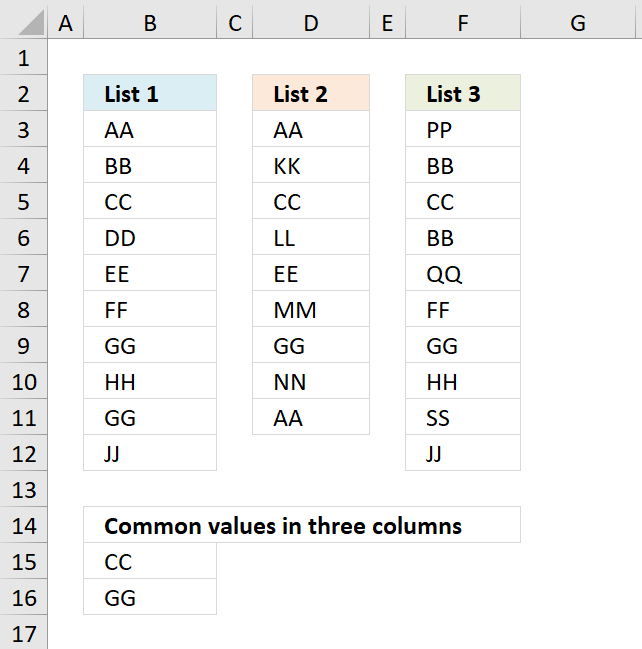Author: Oscar Cronquist Article last updated on November 26, 2018Array formula in B15:

=INDEX(\$B\$3:\$B\$12, MATCH(0, COUNTIF(\$B\$14:B14, \$B\$3:\$B\$12)+IF(((COUNTIF(\$D\$3:\$D\$11, \$B\$3:\$B\$12)>0)+(COUNTIF(\$F\$3:\$F\$12, \$B\$3:\$B\$12)>0))=2, 0, 1), 0))

Copy cell B15 and paste it to cells below as far as necessary.

To enter an array formula, type the formula in a cell then press and hold CTRL + SHIFT simultaneously, now press Enter once. Release all keys.

The formula bar now shows the formula with a beginning and ending curly bracket telling you that you entered the formula successfully. Don't enter the curly brackets yourself.

### Explaining formula in cell B15

#### Step 1 - Prevent duplicates in the list

The COUNTIF function counts values based on a condition or criteria.  The first argument \$B\$14:B14 expands as the cell is copied to cells below. This makes the formula aware of displayed values above the current cell.

COUNTIF(\$B\$14:B14, \$B\$3:\$B\$12)

becomes

COUNTIF("Common values in three columns", {"AA"; "BB"; "CC"; "DD"; "EE"; "FF"; "GG"; "HH"; "GG"; "JJ"})

and returns

{0;0;0;0;0;0;0;0;0;0}

#### Step 2 - Find position of value in array

The MATCH function returns a number representing the position of a value in a list.

MATCH(0,COUNTIF(\$B\$14:B14,\$B\$3:\$B\$12)+(((COUNTIF(\$D\$3:\$D\$11,\$B\$3:\$B\$12)>0)+(COUNTIF(\$F\$3:\$F\$12,\$B\$3:\$B\$12)>0))<>2),0)

becomes

MATCH(0,C{0;0;0;0;0;0;0;0;0;0}+((({TRUE;FALSE;TRUE;FALSE;TRUE;FALSE;TRUE;FALSE;TRUE;FALSE})+({FALSE;TRUE;TRUE;FALSE;FALSE;TRUE;TRUE;TRUE;TRUE;TRUE}))<>2),0)

becomes

MATCH(0,{1;1;0;1;1;1;0;1;0;1},0)

and returns 3.

#### Step 3 - Return value

The INDEX function returns a value based on a row and column number.

INDEX(\$B\$3:\$B\$12, MATCH(0, COUNTIF(\$B\$14:B14, \$B\$3:\$B\$12)+IF(((COUNTIF(\$D\$3:\$D\$11, \$B\$3:\$B\$12)>0)+(COUNTIF(\$F\$3:\$F\$12, \$B\$3:\$B\$12)>0))=2, 0, 1), 0))

becomes

INDEX(\$B\$3:\$B\$12, 3)

and returns "CC" in cell B15.